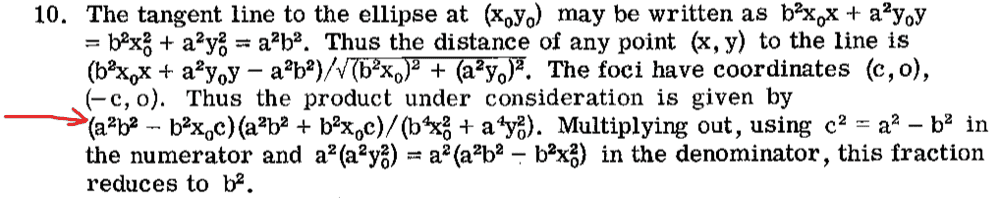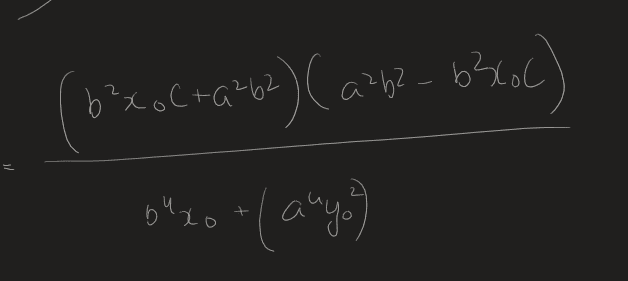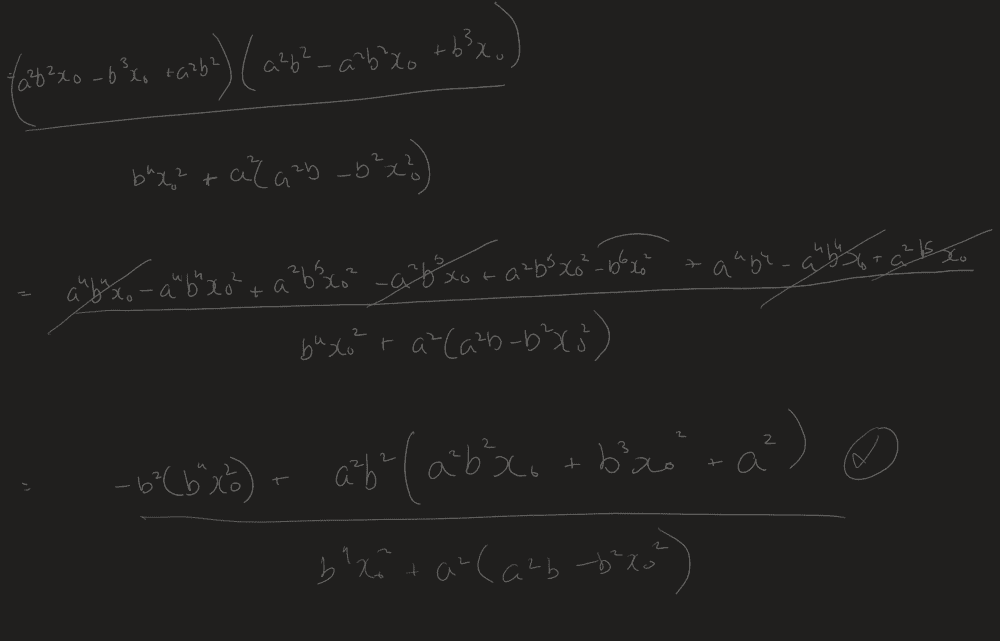# Product of distances from foci to any tangent of an ellipse

Summary:
Show that the product of the distances from the foci to any point of any tangent to an ellipse is ##b^2##
where b is the semi-minor axis.
As part of the final stage of a problem, there is some algebraic manipulation to be done (from the solution manual):But I'm getting lost somewhere:Also a bit of general advice needed: This is part of a self-study Calculus course, and I often have difficulty with bigger algebraic manipulations like this. I often feel if I made a deviation at some point, I will never be able to get to the solution—and I am assuming I need to go through all the possible ways before I have my solution. When in such a situation, I peek at the answer and force the solving into the direction of the solution. Is this bad practice? I feel I shouldn't be spending inordinate amounts of time on algebra in a calculus course.

mfb
Mentor
As written, the statement is clearly wrong. Every point outside the ellipse is element of two tangents, including points that are 1000 b away from the ellipse. What they mean is the distance between the foci and the tangent, not arbitrary points on the tangent.

I don't understand how you get from your first image to the first equation in the second image. Did you swap c <-> c2? It's usually better to simplify first before you plug in things that make the equations longer.
Getting algebra right is an important skill everywhere, so practicing it is a good idea. There are many ways to get to the solution, but a wrong step somewhere will throw off the approach every time. Sometimes it's clear from the wrong answer where things went wrong, sometimes it is not.

You can use LaTeX to write equations here, by the way:
$$\frac{a^2b^2x_0}{b^2+c^2}$$

•ElectronicTeaCup
Thank you for your reply. I notice now that not only did I write the equation incorrectly, (wrote ##b^{4} x_{0}## instead of ##b^{4} x^2_{0}##) I incorrectly substituted ##c^2## for ##c##Thank you for your advice, I have this misconception that algebra is unimportant in the computer age and keep avoiding algebraic mistakes by abandoning questions that involve any algebra I find difficult. I will do better, and stick to it.